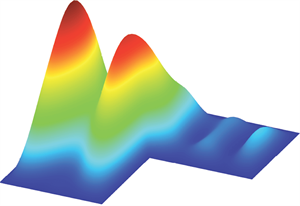# Numerical AnalysisNumerical Analysis research at Birmingham covers a wide range of areas related to the design, analysis and implementation of robust and accurate numerical algorithms for solving mathematical problems coming from real-life applications.

These include numerical methods for partial differential and boundary integral equations, dynamical systems,numerical methods for uncertainty quantification, numerical linear algebra, approximation theory, algorithms for large-scale problems, and development of research software.

The applications of interest include problems in computational fluid dynamics, plasma dynamics and ecology,electromagnetics, linear elasticity, fuel cell modelling, topology optimization, financial modelling and biological fluid mechanics.

Numerical Analysis interacts closely with Optimization, for example organising the biannual IMA Conference on Numerical Linear Algebra and Optimization since 2007, and collaborates with Mathematical Biology .

There exist collaborative links with the following institutions:

• Brunel University
• University of Leicester
• University of Manchester
• University of Oxford
• Rutherford Appleton Laboratory
• ETH Zurich (Switzerland)
• Pontificia Universidad Católica de Chile (Chile)
• Université de Valenciennes (France)

The research interests of the members of the group are given below.

## Dr Alex Bespalov

### Senior Lecturer

Numerical solution of partial differential and boundary integralequations; numerical methods for uncertainty quantification; finite element and boundary element methods; applications to electromagnetics,linear elasticity, and fluid dynamics.

Profile

## Dr Arnaud Lionnet

### Lecturer

Dr Arnaud Lionnet is a Lecturer in Mathematical Statistics. Broadly speaking, he works in probability, numerical methods and mathematical finance and economics.

Profile

## Dr Daniel Loghin

### Lecturer

Numerical linear Algebra, Iiterative methods for linear and nonlinear systems, finite element methods, computational fluid dynamics.

Profile

## Dr Natalia Petrovskaya

### Senior Lecturer

Applied numerical analysis and computer simulation of complex physical and engineering problems. Expert in computational mathematics. The design and exploration of new numerical methods. Examples include computational plasma dynamics, computational aerodynamics and computational ecology.

Profile

## Dr Feng Xu

### Research Fellow

Uncertainty quantification, fluid-structure interaction and thin-film flow

Profile

## Dr Xiaocheng Shang

### Lecturer

Xiaocheng’s primary research interests lie in the optimal design of numerical methods for stochastic differential equations with a strong emphasis on applications ranging from computational mathematics, statistics, physics, to data science.

Profile